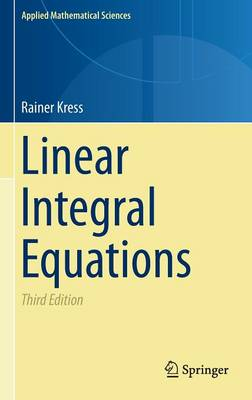•# Linear Integral Equations - Applied Mathematical Sciences 82 (Hardback)

(author)
£74.99
Hardback 412 Pages / Published: 06/12/2013
• We can order this from the publisher

Usually dispatched within 15 working days

This book combines theory, applications, and numerical methods, and covers each of these fields with the same weight. In order to make the book accessible to mathematicians, physicists, and engineers alike, the author has made it as self-contained as possible, requiring only a solid foundation in differential and integral calculus. The functional analysis which is necessary for an adequate treatment of the theory and the numerical solution of integral equations is developed within the book itself. Problems are included at the end of each chapter.

For this third edition in order to make the introduction to the basic functional analytic tools more complete the Hahn-Banach extension theorem and the Banach open mapping theorem are now included in the text. The treatment of boundary value problems in potential theory has been extended by a more complete discussion of integral equations of the first kind in the classical Holder space setting and of both integral equations of the first and second kind in the contemporary Sobolev space setting. In the numerical solution part of the book, the author included a new collocation method for two-dimensional hypersingular boundary integral equations and a collocation method for the three-dimensional Lippmann-Schwinger equation. The final chapter of the book on inverse boundary value problems for the Laplace equation has been largely rewritten with special attention to the trilogy of decomposition, iterative and sampling methods

Reviews of earlier editions:

"This book is an excellent introductory text for students, scientists, and engineers who want to learn the basic theory of linear integral equations and their numerical solution."

(Math. Reviews, 2000)

"This is a good introductory text book on linear integral equations. It contains almost all the topics necessary for a student. The presentation of the subject matter is lucid, clear and in the proper modern framework without being too abstract." (ZbMath, 1999)

Publisher: Springer-Verlag New York Inc.
ISBN: 9781461495925
Number of pages: 412
Weight: 7627 g
Dimensions: 235 x 155 x 24 mm
Edition: 3rd ed. 2014

MEDIA REVIEWS

"The book contains 18 well presented chapters with an extensive list of 249 references. ... It also contains almost all the topics necessary for a student. The presentation of the subject matter is lucid, clear, and in the proper modern framework. ... It is recommended for study to students, teachers, and all others who are interested in the development of this useful, and live area of mathematics." (K. C. Gupta, zbMATH 1328.45001, 2016)

"The book being reviewed is the third edition of a well received one on integral equations which combines theory, applications and also numerical methods. ... the book is more suitable for graduate students. The writing is lucid and clear, and the presentation is excellent. ... the book should appeal not just to mathematicians, but also to scientists and engineers who wish to learn the theory of integral equations, the methods used to solve them, and their applications." (Peter Shiu, The Mathematical Gazette, Vol. 99 (544), March, 2015)